# Polygons – 2 Dimensional Shapes Not Exceeding Octagon | Lines of Symmetry Primary 6 (Basic 6) Term 3 Week 4 Mathematics

### MATHEMATICS

THIRD TERM

WEEK 4

PRIMARY 5

THEME –  GEOMETRICAL SHAPES

PREVIOUS LESSON – Meaning and Types of Lines, Angles and Bearing Primary 5 – Term 3 Week 2 and Primary 6 Term 3 Week 3 Mathematics

### LEARNING AREA

1. Introduction

2. Meaning, Types and Properties of Triangles

3. Meaning, Types and Properties of Quadrilateral

4. Meaning, Types and Properties of Polygons

5. Lines of Symmetry

6. Lesson Evaluation and Weekly Assessment (Test)

### LEARNING OBJECTIVES

By the end of the lesson, most pupils should have attained the following objectives -to

1. identify types and state basic properties of triangles.

3. draw a line of symmetry.

### ENTRY BEHAVIOUR

The pupils can identify and describe shapes like triangle, square, rectangle and circle.

### INSTRUCTIONAL MATERIALS

The teacher will teach the lesson with the aid of chart showing plane shapes.

### METHOD OF TEACHING

Choose a suitable and appropriate methods for the lessons.

Note – Irrespective of choosing methods of teaching, always introduce an activities that will arouse pupil’s interest or lead them to the lessons.

### REFERENCE MATERIALS

1. Scheme of Work

2. 9 – Years Basic Education Curriculum

3. Course Book

4. All Relevant Material

5. Online Information

### LESSON 1 – POLYGONS

ACTIVITY 1 – INTRODUCTION

A polygon is a two dimensional plane figure with three or more sides (straight lines) and angles.

There are different types of polygon,

1. Triangles

3. Pentagon

4. Hexagon

5. Heptagon

6. Octagon

7. Nonagon

8. Decagon

ACTIVITY 2 – TRIANGLES

Triangle is a 3 sided plane figure or shape with sum of angles equal to 180°.

CHARACTERISTICS (PROPERTIES) OF TRIANGLE

A triangle has following properties,

1. Three sides

2. Three angles

3. Three vertices.

4. The sum of the inside angels equal to 180°.

ACTIVITY 3 – TYPES OF TRIANGLES

The major types of triangles are as follows:

1. Equilateral Triangle

2. Isosceles Triangle

3. Scalene Triangle

1. EQUILATERAL TRIANGLE

An equilateral triangle with all the three sides and angles equal.

Each of the angle is a 60°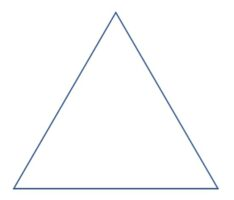2. ISOSCELES TRIANGLE

Isosceles triangle any triangle with two equal sides and two equal angles.

Each of the angle is acute angle, that’s less than 90°.3. SCALENE TRIANGLE

A Scalene triangle is any triangle with unequal sides and angles.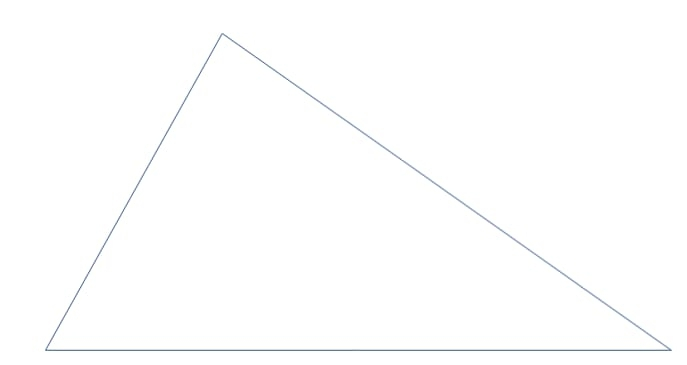There are three types of scalene triangle,

• Right-angled scalene triangle with one of the angle at 90°.
• Acute scalene triangle with all three angles less than 90°.
• Obtuse scalene triangle with one of the angle greater than 90°.

CLASS EXERCISE ON TRIANGLES

1. What is a triangle.

2. State 3 types of triangles.

3. Explain the following,

• Equilateral triangle
• Isosceles triangle
• Scalene triangle

4. Draw the 3 types of triangle.

5. Describe the following,

• Right-angled scalene
• Acute scalene triangle
• Obtuse scalene triangle

6. What is the sum of angles in a triangle?

ACTIVITY 1 – INTRODUCTION TO QUADRILATERALS

Quadrilateral is a four sided plane figure or shape with sum of angles equal to 360°.1. Four sides

2. Four angles

3. Four vertices.

4. The sum of the inside angels equal to 360º.

ACTIVITY 2 – TYPES OF QUADRILATERAL

There are different types of quadrilateral are as follows:

1. Square

2. Rectangle

3. Rhombus

4. Parallelogram

5. Trapezium

1. SQUARE

A square is any quadrilateral with four equal sides and and equal angles.

Each of the angle is at 90º.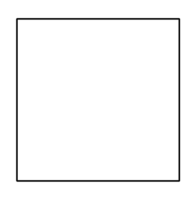2. RECTANGLE

A rectangle is any quadrilateral with two opposite sides equal and equal angles.

Each of the angle is at 90º.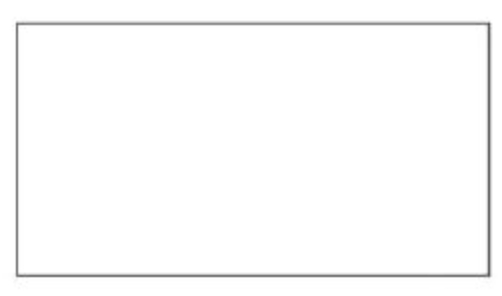3. RHOMBUS

Rhombus is any quadrilateral with four equal parallel sides and equal opposite angles.

Rhombus is not the same with a square.

4. PARALLELOGRAM

A parallelogram is any quadrilateral with equal opposite sides parallel and equal opposite angles.

5. TRAPEZIUM

Trapezium is any quadrilateral with two unequal parallel sides and two non parallel sides.

There are 3 types of trapezium,

• Isosceles trapezium with two equal sides.
• Scalene trapezium with no equal sides.
• Right trapezium with two right angles.

ACTIVITY 3 – CLASS EXERCISE

2. State 4 types of quadrilateral?

3. Draw 3 types of quadrilateral?

4. Differentiate between square and rectangle.

5. describe the following:

• Right angle trapezium
• Isosceles trapezium
• Scalene trapezium

7. Rhombus is not a square. Discuss.

8. Each type of quadrilateral is a complete parallelogram except _________.

9. What is the sum of angles in a quadrilateral?

### LESSON 3 – POLYGONS MORE THAN 4 STRAIGHT LINES

ACTIVITY 1 – PENTAGON

Pentagon is a polygon with five equal sides and five equal angles.

Each of the angle is 108° with total of 540°.

ACTIVITY 2 – HEXAGON

Hexagon is a polygon with six equal sides and six equal angles.

Each of the angle is 120° with total of 720°.

ACTIVITY 3 – HEPTAGON

Heptagon is a polygon with seven equal sides and seven equal angles.

The sum of the angles is 900°.

Each of the angle is approximately 128.57°.

ACTIVITY 4 – OCTAGON

Octagon is a polygon with eight equal sides and eight equal angles.

Each of the angle is 135° with total of 1080°.

ACTIVITY 5 – NONAGON

Octagon is a polygon with nine equal sides and nine equal angles.

Each of the angle is 140° with total of 1260°.

ACTIVITY 6 – DECAGON

Octagon is a polygon with ten equal sides and ten equal angles.

Each of the angle is 144° with total of 1440°.

CLASS EXERCISE – POLYGONS

1. What is a polygon?

2. State 5 types of polygon you know.

3. Polygon is any shape with 3 or more sides. True or False

4. State all the polygon with 5 or more sides.

5. What is the number of sides and sum of angles in each of the following:

• Triangle
• Pentagon
• Hexagon
• Heptagon
• Nonagon
• Decagon

6. Draw the shape of the following:

• Triangle
• Pentagon
• Hexagon
• Heptagon
• Nonagon
• Decagon

### LESSON 4 – LINES OF SYMMETRY

ACTIVITY 1 – INTRODUCTION

A line of symmetry is a line that a shape exactly into two.

For example,

If you fold a shape such a square into two and both parts would match exactly, the line in between is known as line of symmetry.

ACTIVITY 2 – FOLDING SHAPES WITH 3 SIDES OR MORE

Teacher organizes pupils in groups or pairs depending on the size of the class to determine the lines of symmetry by folding or cutting.

1. Triangles

There are 3 major types of triangles,

Equilateral triangle has 3 lines of symmetry.

Isosceles triangle has one line of symmetry.

Scalene Triangle has zero line of symmetry.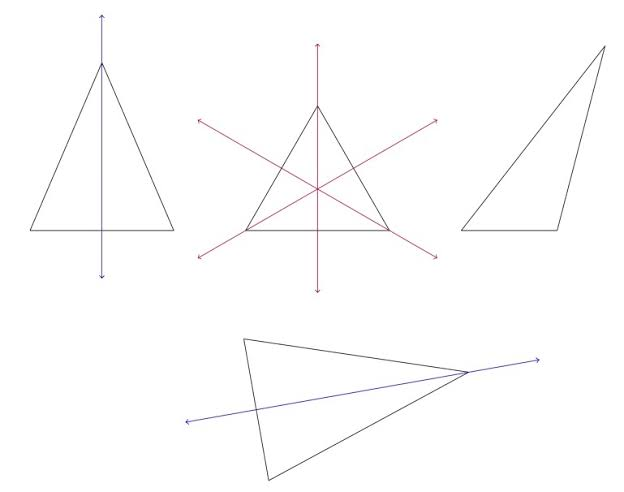There are different types of quadrilateral are as follows:

1. Square has 4 lines of symmetry.

2. Rectangle has 2 lines of symmetry.

3. Rhombus has 2 lines of symmetry.

4. Parallelogram has no line of symmetry.

5. Trapezium has no line of symmetry.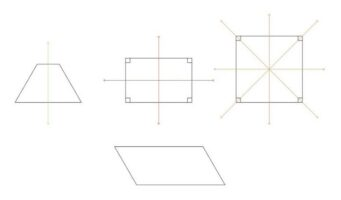3. Pentagon

Polygon has 5 lines of symmetry.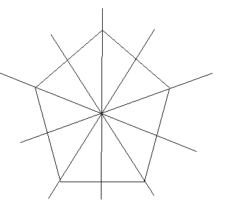4. Hexagon

Hexagon has 6 Lines of symmetry.5. Heptagon

Heptagon has 7 lines of symmetry.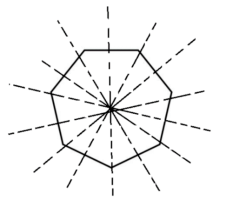6. Octagon

Octagon has 8 lines of symmetry.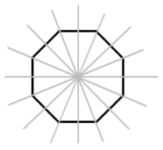7. Nonagon

Nonagon has 9 lines of symmetry.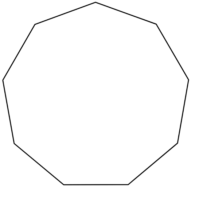8. Decagon

Decagon has 10 lines of symmetry.LESSON 5 – REVISION AND WEEKLY ASSESSMENT

As stated in performance objectives or lesson evaluation.

### PRESENTATION

To deliver the lesson, the teacher adopts the following steps:

1. To introduce the lesson, the teacher revises the previous lesson. Based on this, he/she asks the pupils some questions;

2. Teacher organizes the pupils in groups or pair depending on the size of the class.

3. Teacher ask the groups or pairs to drawing the following shapes – triangle, square, rectangle and circle.

4. Teacher uses the pupils drawing to introduce the lesson and leads a discussion on the meaning and types of plane shapes.

5. Teacher displays different types of triangles and guides pupils to measure and record the sides and angles of each types of triangle.

6. Teacher uses the activity 5 to discuss the meaning and types of triangles with their properties.

7. Teacher displays different types of quadrilateral and guides pupils to measure and record the sides and angles of each type of quadrilateral.

8. Teacher uses the activity 5 to discuss the meaning and types of quadrilateral with their properties.

9. Teacher displays different types of polygon and guides pupils to compare the number of sides, measure and record the sides and angles of each type of polygon.

10. Teacher uses the activity 5 to discuss the meaning and types of polygon with their properties.

11. Teacher displays both sample  and chart of all polygons.

12. Teacher guides the group or pairs to fold and cut each shape into two.

Pupil’s Activities – Carefully fold or cut each shape into two and compare.

13. Teacher uses pupil’s activity in steps 12 to introduce and discuss the lines of symmetry.

Pupil’s Activities – Identify the number of lines of symmetry on plane shapes.

14. Teacher summarizes the lesson on the board with appropriate evaluation.

Pupil’s Activities – Participate actively in the summary of the lesson and write as instructed.

### CONCLUSION

To conclude the lesson for the week, the teacher revises the entire lesson and links it to the following week’s lesson.

### LESSON EVALUATION

1. explain the meaning of plane shape with examples.

2. state 5 types of plane shapes.

3. what is a triangle?

4. list and explain each types of triangles.

6. state and explain each types of quadrilateral.

7. what is a polygon?

8. write short note on the following:

• pentagon
• hexagon
• heptagon
• nonagon
• decagon

9. state the number of lines of symmetry for each of the following:

• triangle